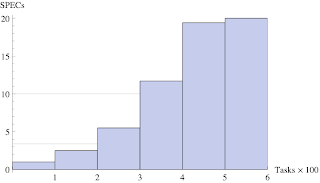## Wednesday, November 19, 2008

### What the Harmonic Mean Means

As I discuss in Chapter 1 of The Practical Performance Analyst, time is the fundamental performance metric. Computer system performance metrics are therefore either direct measures of time, e.g, seconds, hours, minutes, or they are rates. All rate metrics have their units of time in the denominator, e.g., GB/s, MIPS, TPS, IOPS.

A conceptual difficulty can arise when we try to summarize a set of performance numbers as a single number; especially if they're rates.The usual approach is to employ the average value to express a single number, but there is more than one way to calculate an average or mean value. In particular, there is the arithmetic mean (A); the one you learnt in school, the geometric mean (G); used in SPEC CINT2006 benchmark ratings (rightly or wrongly), and the lesser-known harmonic mean (H). For example, the harmonic mean of three quantities: $a, b$, and $c$ is: \begin{equation} H = \dfrac{3}{\frac{1}{a} + \frac{1}{b} + \frac{1}{c}} = \dfrac{3 \, abc}{a + b + c} \end{equation} We discuss each of these means at length in the GDAT class. We also point out that the magnitude of these means are always ranked according to: H < G < A, when applied to the same data. In other words, except for special cases, H is always the least of them and A is always the greatest.

That said, almost all of us (including me) promptly ignore these distinctions in practice; especially when it comes to the application of the harmonic mean. One reason for this avoidance is the unintuitive nature of the harmonic mean. However, when it comes to averaging rate metrics, we really do need to apply the harmonic mean. Here's why.

All averaging involves addition. Because rate metrics are inverted-time quantities, adding rates is analogous to adding fractions with different denominators. For example, you can't add 5/3 + 1/5 by simply adding the top numbers together and the bottom numbers together (Well, you can but those are called Farey fractions, and that's a whole different topic). When adding fractions that have different denominators (in ordinary arithmetic), you need to find the Lowest Common Denominator or LCD. No doubt you remember from school, how unintuitive the LCD notion was. We need to do the same kind of thing to determine the harmonic mean, and you can see that going on in the equation above.

Because the harmonic mean is unintuitive, it is hard to see how to apply it in practical situations. So, now I'm going to present two examples where the harmonic mean is absolutely necessary: (1) variable-speed processors and (2) load balancing servers.

Let's start with a very simple example involving just two speed settings and then I'll generalize it.

1. Variable-speed Processing

1. Suppose we process 1000 writes at 400 IOPS followed by 1000 reads at 600 IOPS. Naturally, the two types of IO operations will take different elapsed times to complete. Overall, the total time to process 2000 IOs is 4.167 seconds. We could ask the question: What average IO rate would allow us to complete 2000 IOs is 4.167 seconds? If we assume the that arithmetic mean (A) of the rates represents the average IO rate, we get: \begin{equation} A = \dfrac{1}{2} (400 + 600) = 500 ~\text{IOPS} \end{equation} But that would result in completing the IOs in just 2000 IOs/500 IOPS = 4 seconds. On the other hand, if we assume the harmonic mean instead, then using the definition above for 2 quantities (i.e., a and b), we find: \begin{equation} H = \dfrac{2}{\frac{1}{400} + \frac{1}{600}} = \dfrac{2 (400 × 600)}{1000} = 480 ~\text{IOPS} \end{equation} and 2000 IOs /480 IOPS = 4.167 seconds; which is correct.

Note that H is computed by adding the inverse IOPS rates. That's so we are effectively averaging time-based quantities (which is the same as inverse rates). From this example, it should be clear that the harmonic mean is the correct type of average to use when it comes to summarizing rates.

2. Now let's generalize this idea to the case where we have a truly variable-speed "green" processor that ramps up its service rate (e.g., as measured by its SPEC value) to meet demand. Otherwise, it cycles at low speed in order to consume less electrical power. The plot below shows the respective processor speed in SPEC (rate) units as it processes 100 tasks in each of 6 increments. The time to process all 600 tasks is 176.88 seconds. The harmonic mean H = 3.39 TPS (lower horizontal line), while the corresponding arithmetic mean A = 10.02 TPS (upper horizontal line); a significant difference! This large difference is a direct consequence of inverting the rates in the harmonic sum, which ends up giving more weight to the first 4 columns in the plot.2. Load Balancing Example

Consider application requests arriving at a rate of λ = 100 TPS to be serviced by 2 application servers; one of which is faster than the other. The fast server handles 60 TPS and takes 10 milliseconds to process each request (on average), while the slow server only handles 40 TPS and takes 20 milliseconds per request. If we measured the utilization of each server, we would find the fast server is 60% busy, while the slower server is 80% busy, i.e., they are unbalanced. What service speed will balance them? To achieve peace and harmony, we compute the harmonic mean of the service times: \begin{equation} S_H = \dfrac{2}{\frac{1}{10} + \frac{1}{20}} = 13.33 ~\text{ms} \end{equation} and split the incoming transactions equally across the 2 servers so that each one gets requests at a rate of $\lambda/2 = 50$ TPS. We can use Little's law $(\rho = \lambda S_H/2)$ to confirm that each server will be balanced at $\rho = 50 ~\text{TPS} \times 0.0133333 ~\text{s} = 0.6667$ or 67% busy.

I'll probably present more on this topic during my Capacity Planning Boot Camp sessions at CMG 2008.